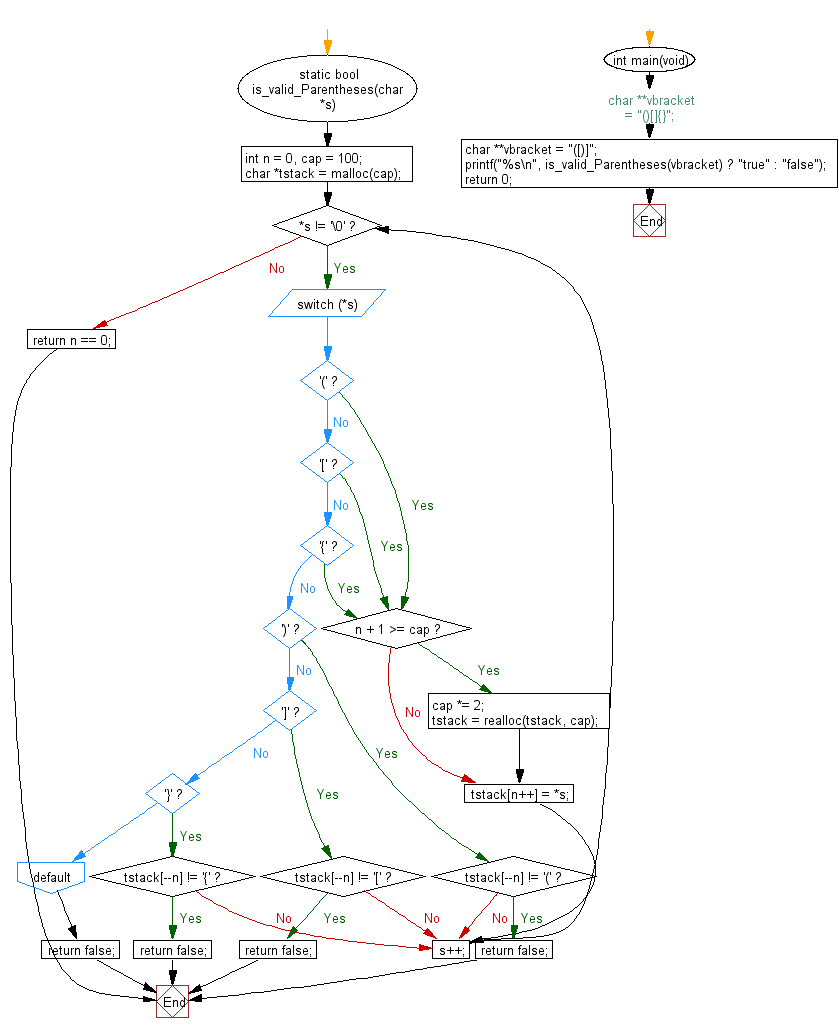﻿ C Program: Check if a given string is valid or not - w3resource# C Exercises: Check if a given string is valid or not

## C Programming Practice: Exercise-10 with Solution

Write a C programming to check if a given string is valid or not, the string contains the characters '(', ')', '{', '}', '[' and ']'. The string is valid if the open brackets must be closed by the same type of brackets and in correct order.

C Code:

``````#include <stdio.h>
#include <stdlib.h>
#include <stdbool.h>
static bool is_valid_Parentheses(char *s)
{
int n = 0, cap = 100;
char *tstack = malloc(cap);

while (*s != '\0') {
switch(*s) {
case '(':
case '[':
case '{':
if (n + 1 >= cap) {
cap *= 2;
tstack = realloc(tstack, cap);
}
tstack[n++] = *s;
break;
case ')':
if (tstack[--n] != '(') return false;
break;
case ']':
if (tstack[--n] != '[') return false;
break;
case '}':
if (tstack[--n] != '{') return false;
break;
default:
return false;
}
s++;
}
return n == 0;
}
int main(void)
{
//char **vbracket = "()[]{}";
char **vbracket = "([)]";
printf("%s\n", is_valid_Parentheses(vbracket) ? "true" : "false");
return 0;
}
``````

Sample Output:

```false
```

Flowchart:## C Programming Code Editor:

What is the difficulty level of this exercise?

Test your Programming skills with w3resource's quiz.

﻿

## C Programming: Tips of the Day

What is the difference between printf() and puts() in C?

puts is simpler than printf but be aware that the former automatically appends a newline. If that's not what you want, you can fputs your string to stdout or use printf.

Ref :https://bit.ly/3f2fwcH